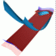Abstract and Contents
Up: 5. Examples
Next: 6. Discussion
Previous: 5.4 The Lorenz system

# 5.5 Normal form of the Hopf-Hopf bifurcation

In the case of a periodic vector field, a three-dimensional stable or unstable manifold can be computed as a two-dimensional manifold of the associated Poincaré map. This is demonstrated here with the three-dimensional stable and unstable manifolds of an invariant torus. The vector field under consideration is the normal form of a double Hopf bifurcation without resonance. Up to third order, the system is given in toroidal coordinates as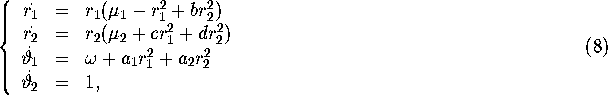whereand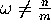for. The unfolding is discussed in [Guckenheimer and Holmes 1983, Chow et al. 1994]. Throughout this section we fix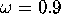,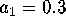and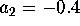.

For b = 2, c = 1 and d = -1, an invariant torus of saddle-type exists in (8) in the entire region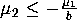and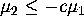. Hence, by taking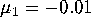and, the associated Poincaré map defined on the section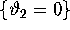has an invariant circle with both a stable and an unstable normal direction. The circle is found by solving. The linear approximation of its stable and unstable manifolds is given by the eigenvectors of this two-dimensional system times the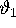-axis.

Starting at distance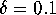on the linear approximation, we compute the stable and the unstable manifolds. We use 50 mesh points on the circle, and maintain a maximal distance of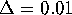between neighboring points. For the bisection error we take. The Poincaré map is obtained by integration of (8) with the fourth-order Runge-Kutta method from [Press et al. 1992].

The result is shown in Figure 17; the colored bands in this picture have width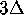, except for the part of the unstable manifold that converges to the circle at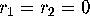. For this part a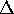-step is too large and the size of each ring is equal to the minimal distance of the inner boundary of this ring to its f-image. In [Guckenheimer and Holmes 1983, Chow et al. 1994] the stable and unstable manifolds are qualitatively sketched in the-plane for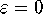. The invariant torus of the vector field is then a fixed point. One branch of the unstable manifold of this fixed point converges to the origin, which is another fixed point. The two branches of the stable manifold are asymptotic to the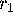- and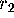-axis, respectively. In-space, both fixed points are invariant tori. Hence, one branch of the unstable manifold of the circle converges to the invariant circle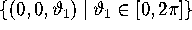. The branches of the stable manifold are symptotic to the- and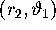-plane, respectively. Note that due to the special form of (8) the manifolds are linear in the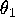direction.

Up: 5. Examples
Next: 6. Discussion
Previous: 5.4 The Lorenz systemAbstract and Contents

Written by: Bernd Krauskopf & Hinke Osinga
Created: May 27 1997 --- Last modified: Fri May 30 19:55:22 1997